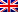Share# Coding Challenge November 2019 For Students @Chiang Mai

## Coding Challenge November 2019 For Students @Chiang Mai

Coding Instructions:
The following German words represent a mathematical formula:
HIER + GIBT + ES = NEUES

Conditions:
– every letter symbolizes one digit [0..9]
– the 10 different letters have to be 10 different digits
– every word is an unsigned integer number (no leading zeros)

Challenge:
– create a PHP class containing a private method that solves the problem mentioned
– “worst case” solution: brute force (1010 iterations = 10,000,000,000 iterations)
– “best case” solution: use mathematical rules to reduce the amount of iterations of the problem (“if then continue”)
– use a counter variable to measure the amount of loops needed to find possible results

```\$iterations = 0;
[loops to assign digits to the letters]
e.g.: H=9;I=8;E=7;R=6;G=5;B=4;T=3;S=2;N=1;U=0
9876 + 5843 + 72 !== 17072  // not a correct solution
\$iterations++
[/loops]
```

– the method should output all results – if there are any – including the amount if iterations needed
– use comments to describe the mathematical rules used to reduce the complexity of the task
– the first place goes to the correct solution having the least iterations

Our Prizes:
1st Prize: Gift Voucher ฿1,000.00 (Central Voucher or Major Voucher or Starbucks Voucher)
2nd  till  4th Prize: Gift Voucher ฿750.00 (Central Voucher or Major Voucher or Starbucks Voucher)
5th  till  10th Prize: Starbucks Voucher ฿300.00

Please send your answer and attached PHP file with a copy of your student ID and mobile phone number for reference via email to: info@data-horizon.com

Competition closes on the 1st of December 2019. The winners will be announced on 2nd of December 2019.